# How to Calculate and Solve for Relationship between Modulus of Elasticity on Volume Fraction Porosity, E | Ceramics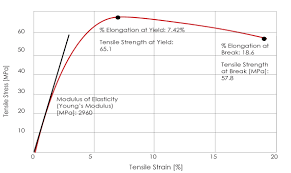The image above represents modulus of elasticity.

To compute for the relationship between modulus of elasticity on volume fraction porosity, two essential parameters are needed and these are Modulus of Elasticity of Non Porous Material (Eo) and Volume Fraction Porosity (P).

The formula for calculating the modulus of elasticity:

E = Eo(1 – 1.9P + 0.9P²)

Where:

E = Modulus of Elasticity
Eo = Modulus of Elasticity of Non Porous Material.
P = Volume Fraction Porosity

Let’s solve an example;
Find the modulus of elasticity when the modulus of elasticity of non porous material is 12 and volume fraction porosity is 22.

This implies that;

Eo = Modulus of Elasticity of Non Porous Material = 12
P = Volume Fraction Porosity = 22

E = Eo(1 – 1.9P + 0.9P²)
E = (12)(1 – 1.9(22) + 0.9(22)²)
E = (12)(1 – (41.8) + 0.9(484))
E = (12)(1 – (41.8) + (435.6))
E = (12)(394.8)
E = 4737.6

Therefore, the modulus of elasticity is 4737.6 Pa.

Nickzom Calculator – The Calculator Encyclopedia is capable of calculating the modulus of elasticity.

To get the answer and workings of the modulus of elasticity using the Nickzom Calculator – The Calculator Encyclopedia. First, you need to obtain the app.

You can get this app via any of these means:

To get access to the professional version via web, you need to register and subscribe for NGN 1,500 per annum to have utter access to all functionalities.
You can also try the demo version via https://www.nickzom.org/calculator

Apple (Paid) – https://itunes.apple.com/us/app/nickzom-calculator/id1331162702?mt=8
Once, you have obtained the calculator encyclopedia app, proceed to the Calculator Map, then click on Materials & Metallurgical under Engineering.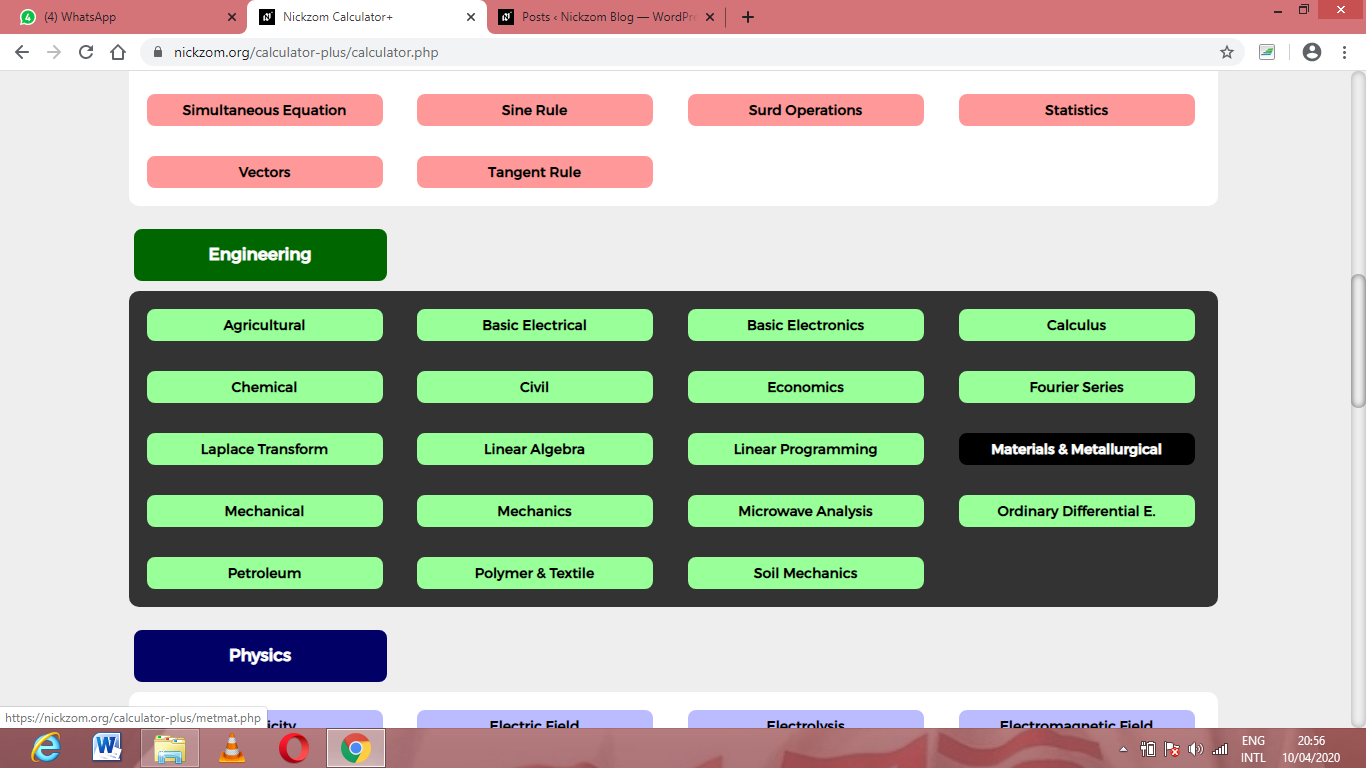Now, Click on Ceramics under Material & Metallurgical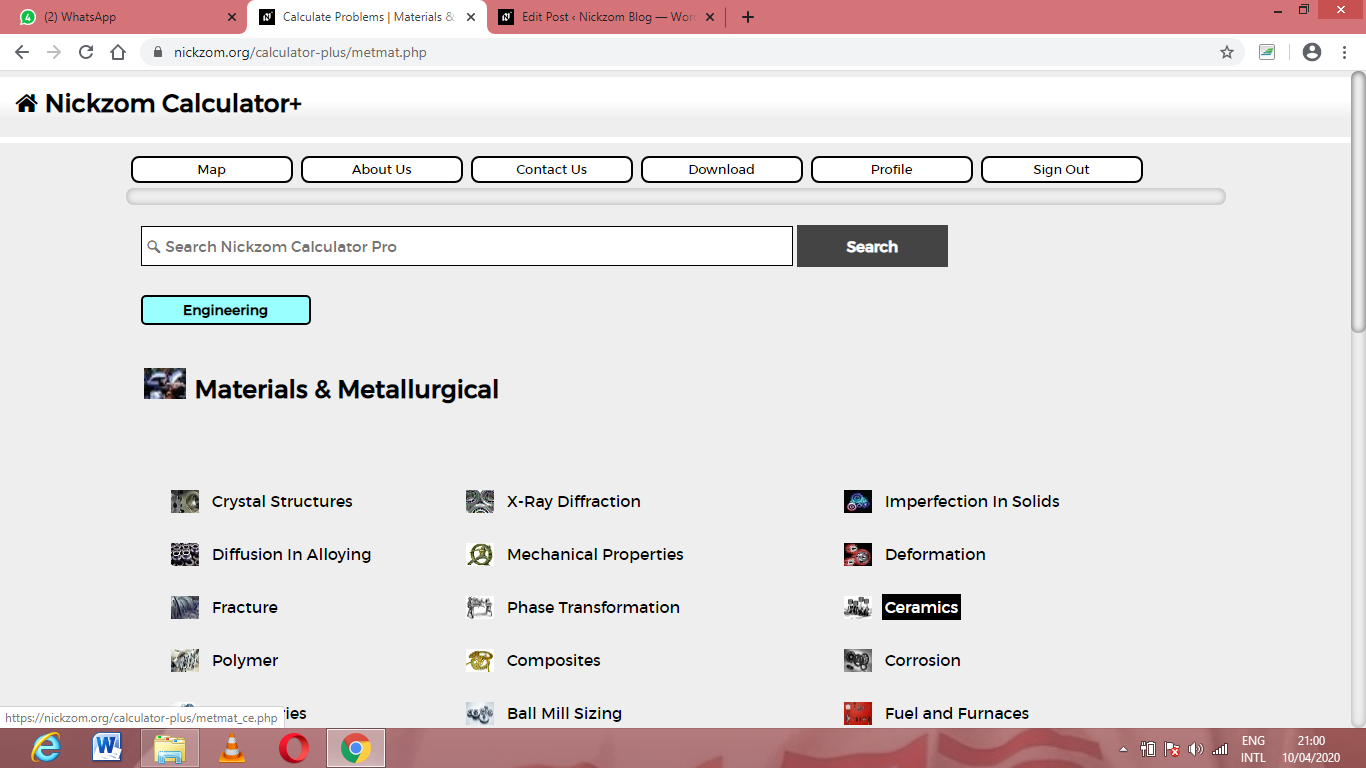Now, Click on Relationship Between Modulus of Elasticity on Volume Fraction Porosity under Ceramics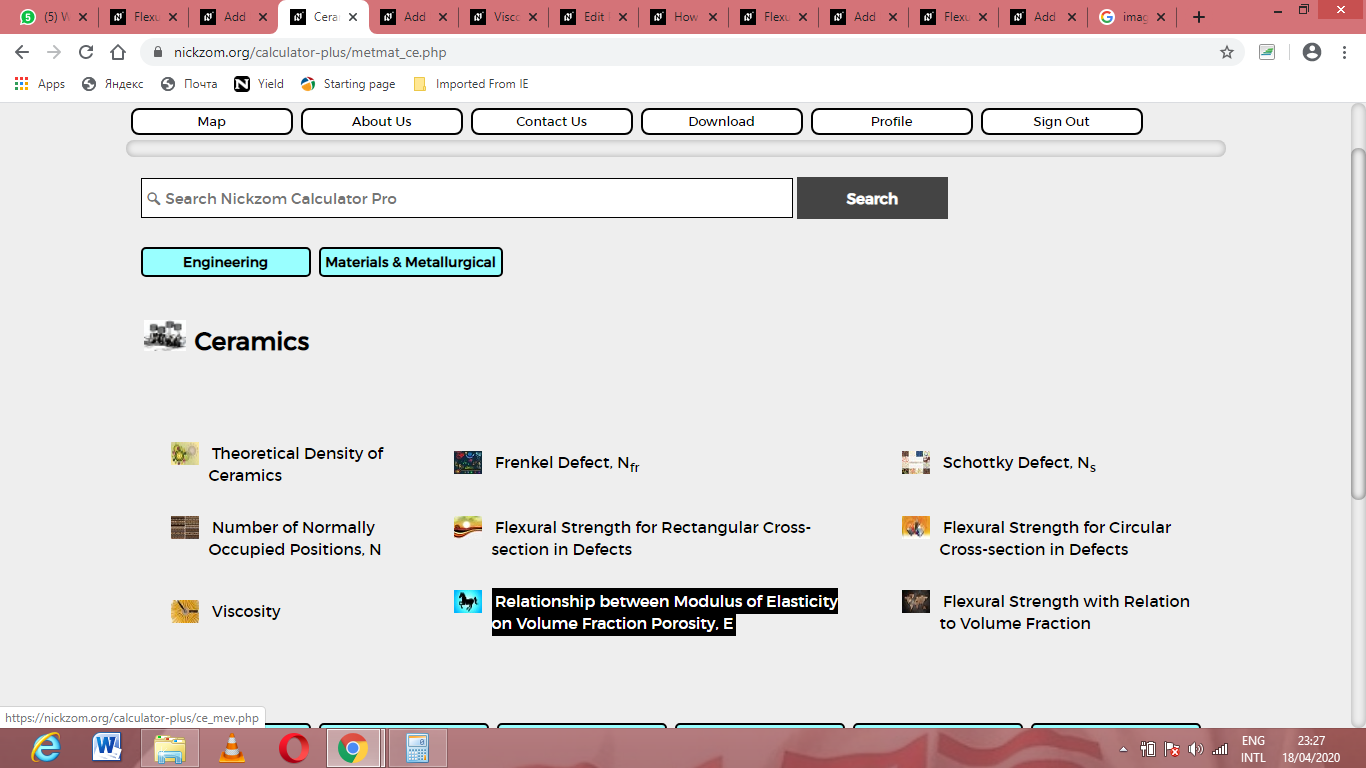The screenshot below displays the page or activity to enter your values, to get the answer for the modulus of elasticity according to the respective parameters which are the Modulus of Elasticity of Non Porous Material (Eo) and Volume Fraction Porosity (P).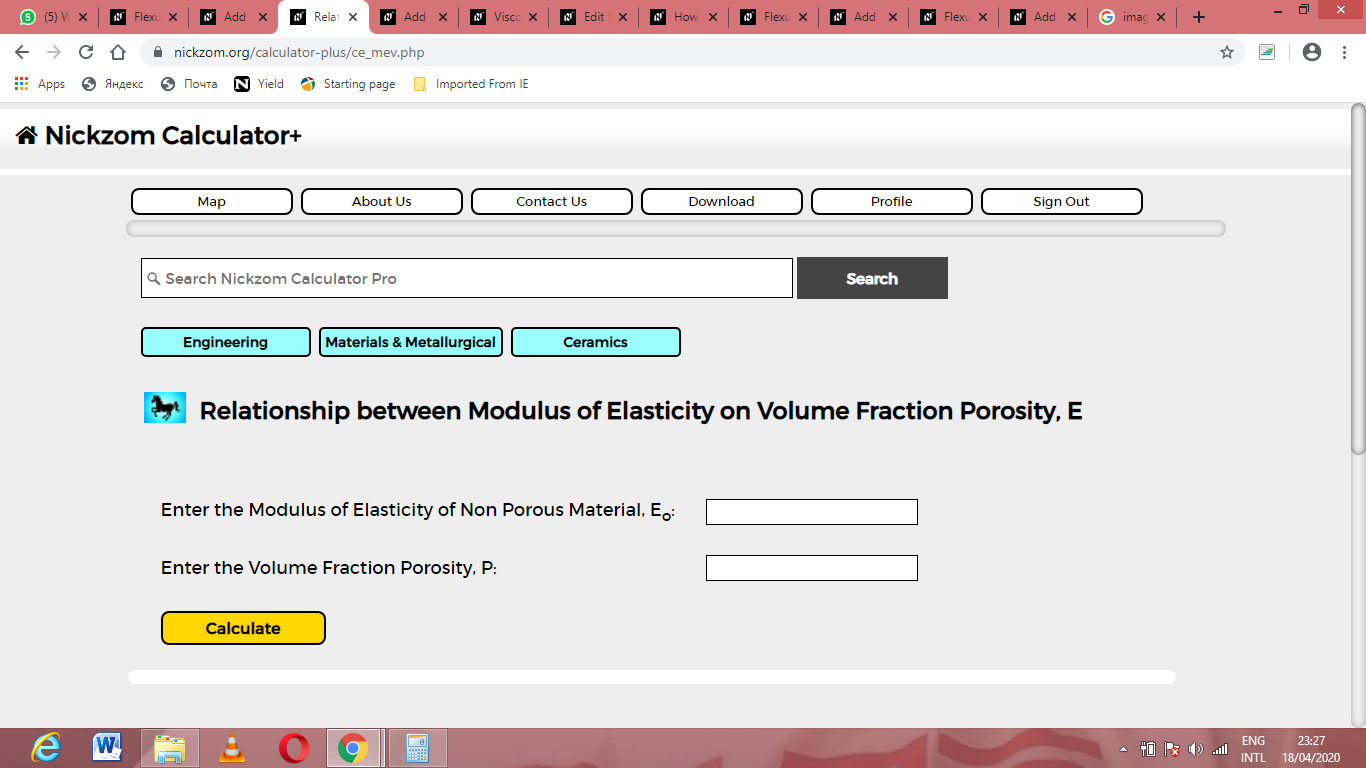Now, enter the values appropriately and accordingly for the parameters as required by the Modulus of Elasticity of Non Porous Material (Eo) is 12 and Volume Fraction Porosity (P) is 22.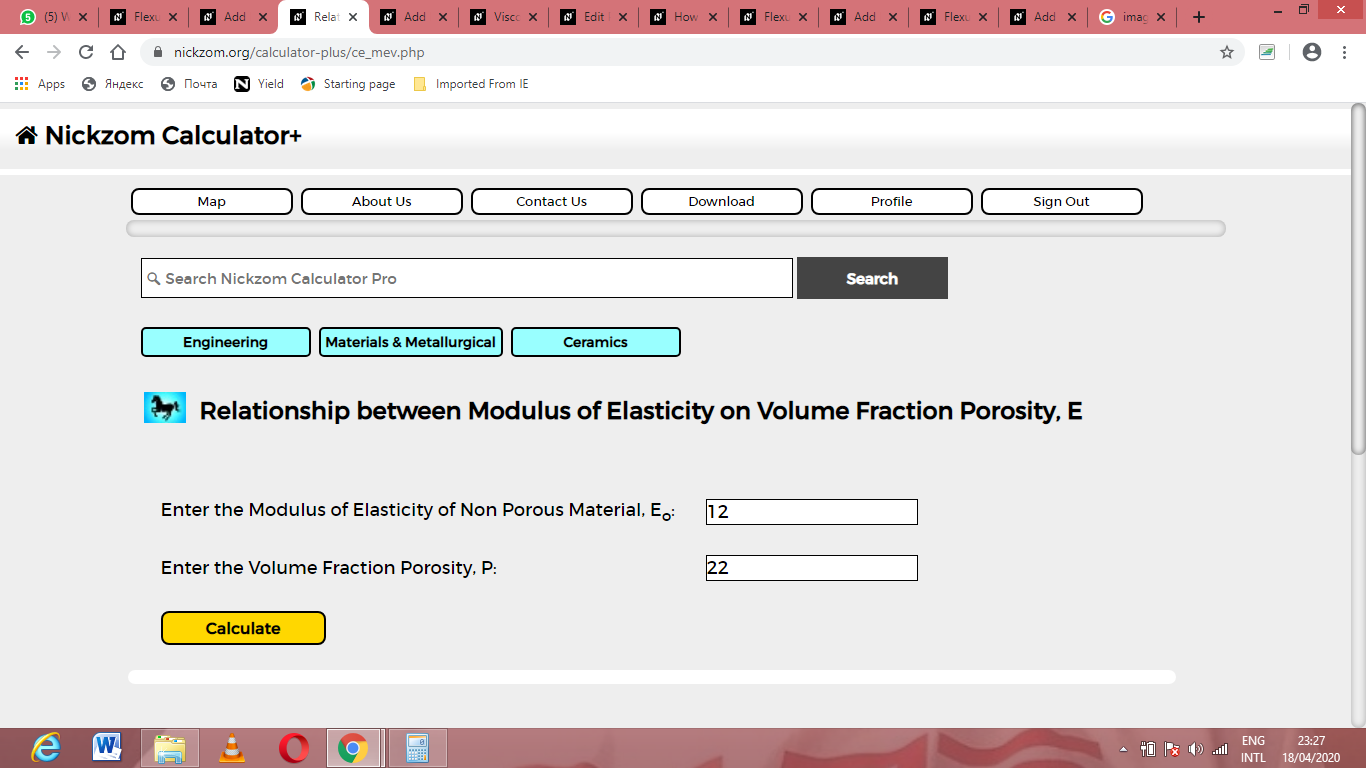Finally, Click on Calculate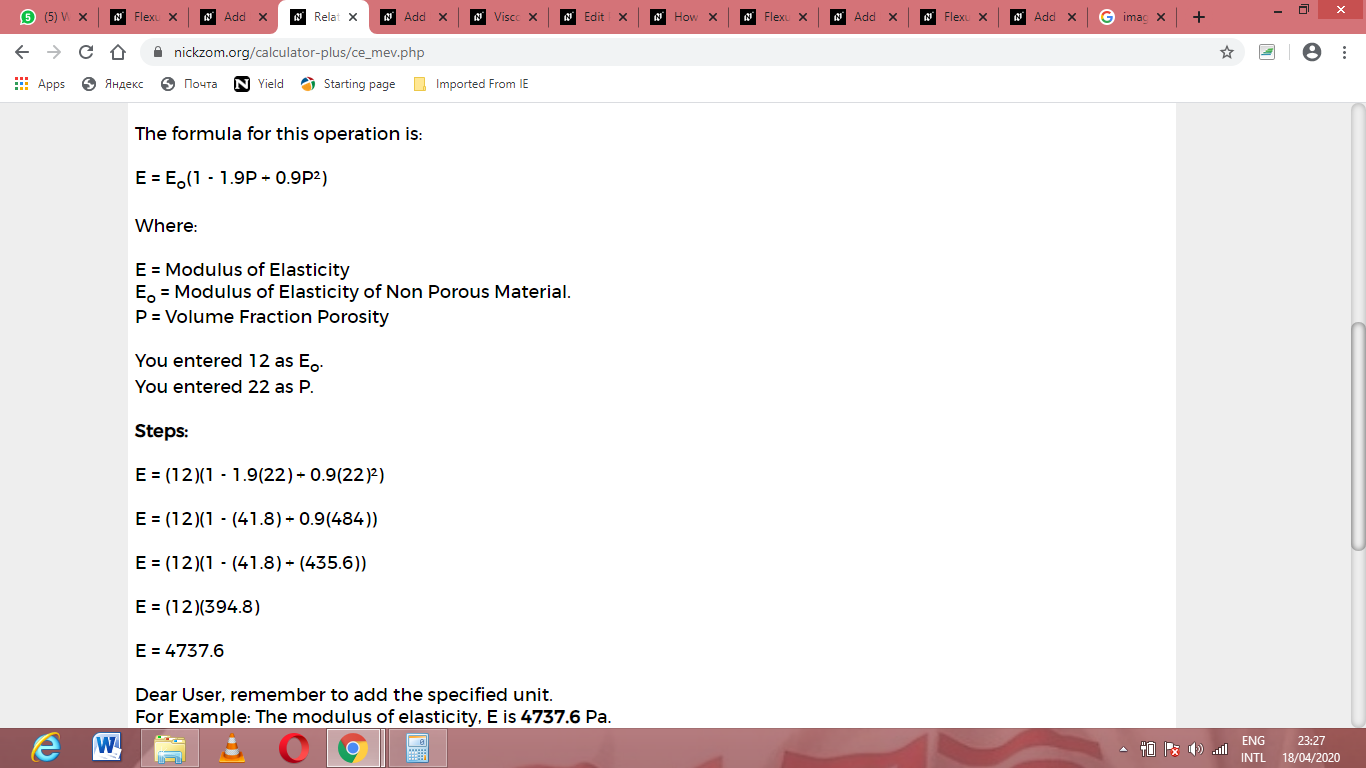As you can see from the screenshot above, Nickzom Calculator– The Calculator Encyclopedia solves for the modulus of elasticity and presents the formula, workings and steps too.